# NCERT Exemplar Solutions for Class 9 Maths Chapter 13 - Surface Areas and Volume

## NCERT Exemplar Solutions Class 9 Maths Chapter 13 – Free PDF Download

NCERT Exemplar Class 9 Mathematics Chapter 13 Surface Areas and Volumes are provided here for students to prepare for exams and make them score well. These exemplars problems and solutions are designed by experts in accordance with the CBSE latest syllabus (2021-2022). The Exemplars Solutions provided for this chapter can be used as a reference tool while solving the exercise questions of Chapter 13 from the NCERT book. Students can also use it for revising the chapter during the exams. The following topics of Surface area and volumes are covered;

• Formulas for Surface Area of a Cuboid and a Cube
• Formulas for Surface Area(curved surface and total surface) of a Right Circular Cylinder
• Curved surface area and total surface area of a cone
• The surface area of a sphere
• Curved Surface Area and Total Surface Area of a Hemisphere
• Volumes of Cuboid, Cylinder, Right Circular Cone, Sphere and Hemisphere.

In this chapter, the students will learn how to calculate the surface area and volume of various shapes like spheres, cylinders, cones, and cuboids. For reference, students can also use online study materials provided by BYJU’S such as exemplar books, notes, NCERT Solutions for Class 9. To understand the question pattern and marking scheme, students should solve sample papers and previous year question papers of Class 9.

## Download PDF of NCERT Exemplar Solutions for Class 9 Maths Chapter 13 Surface Areas and Volumes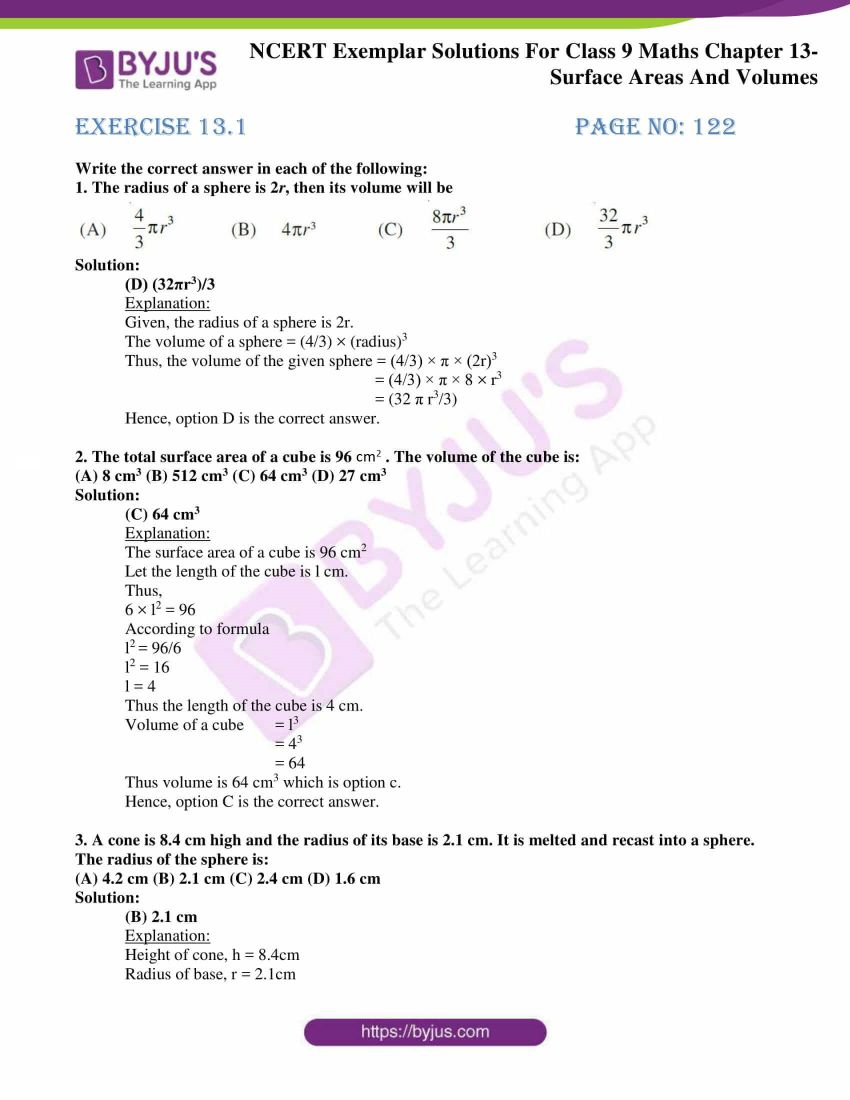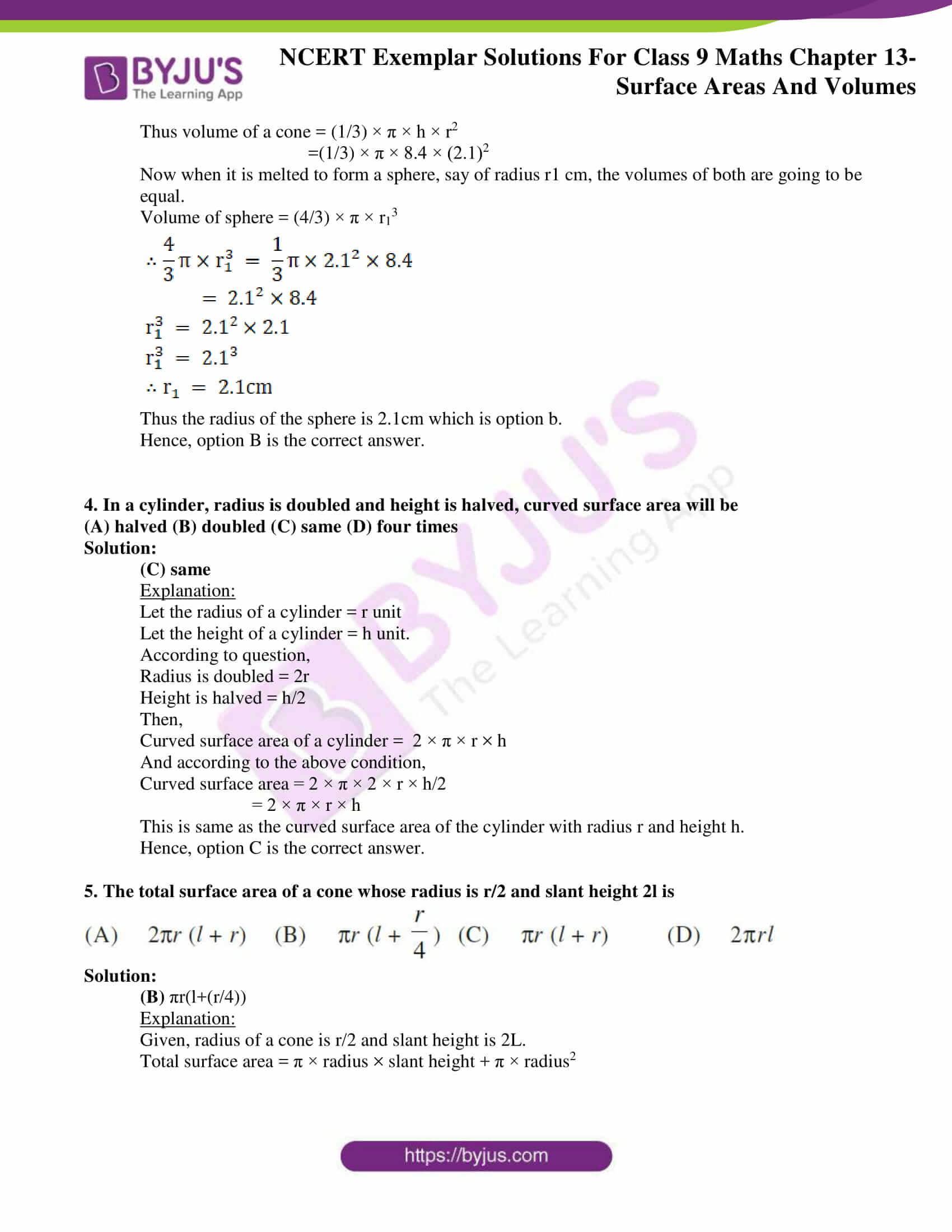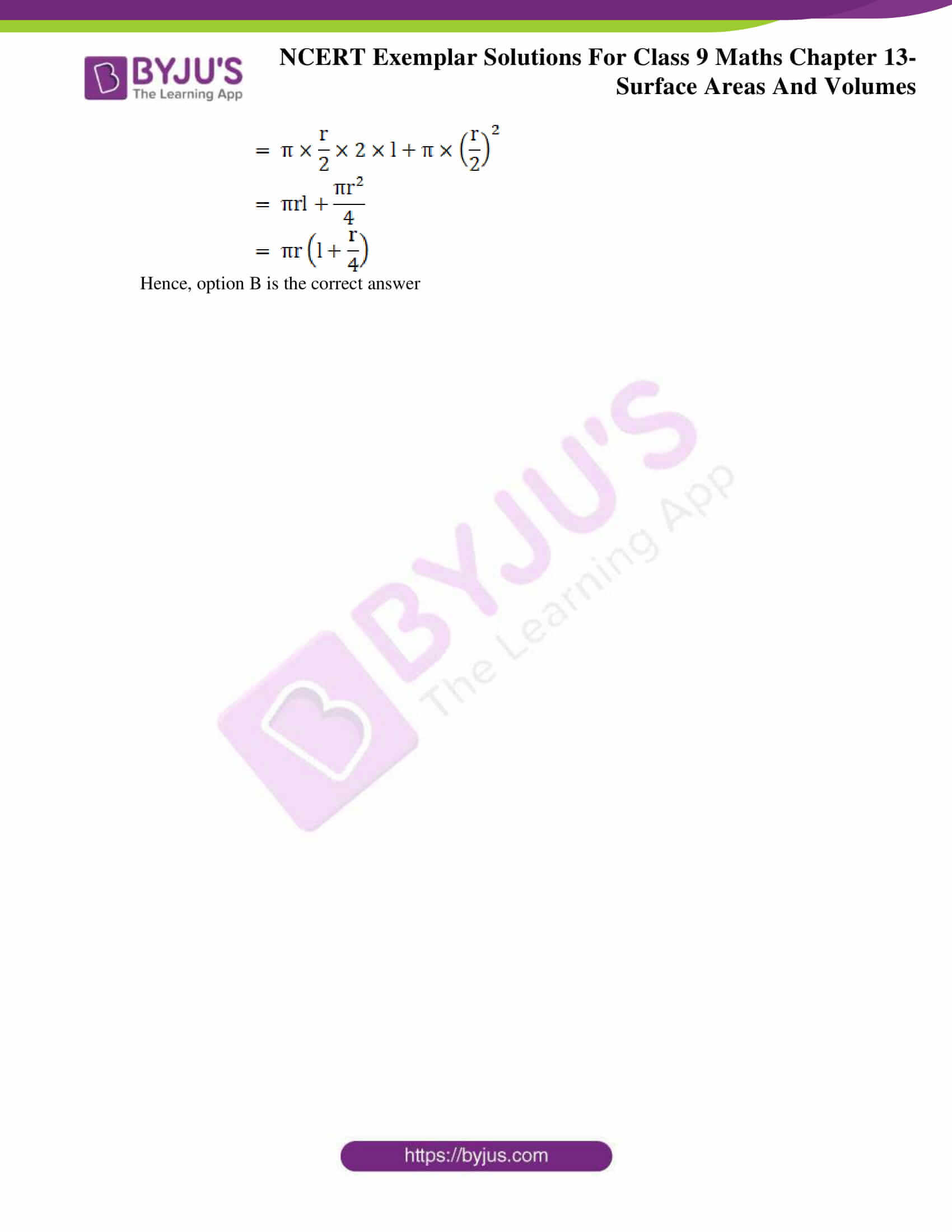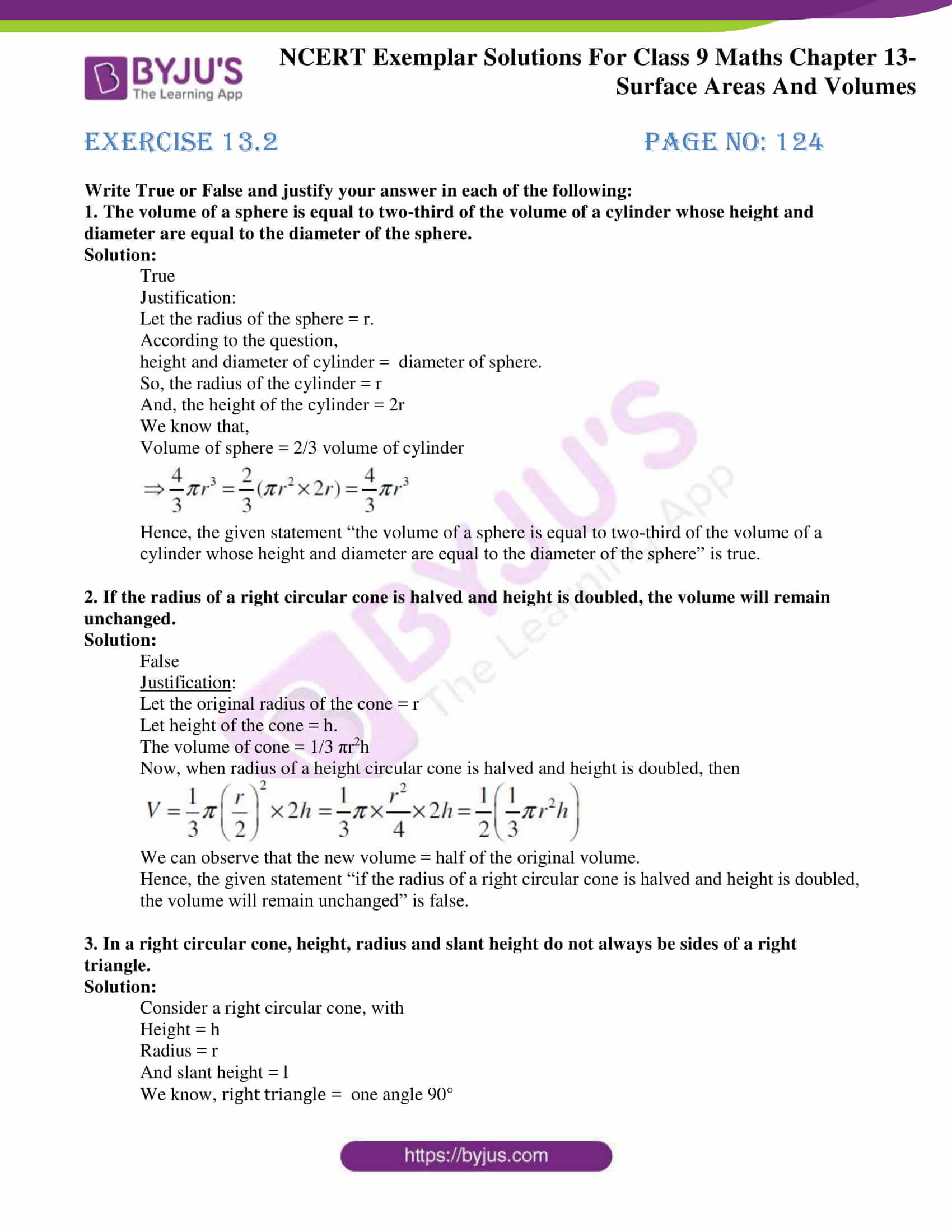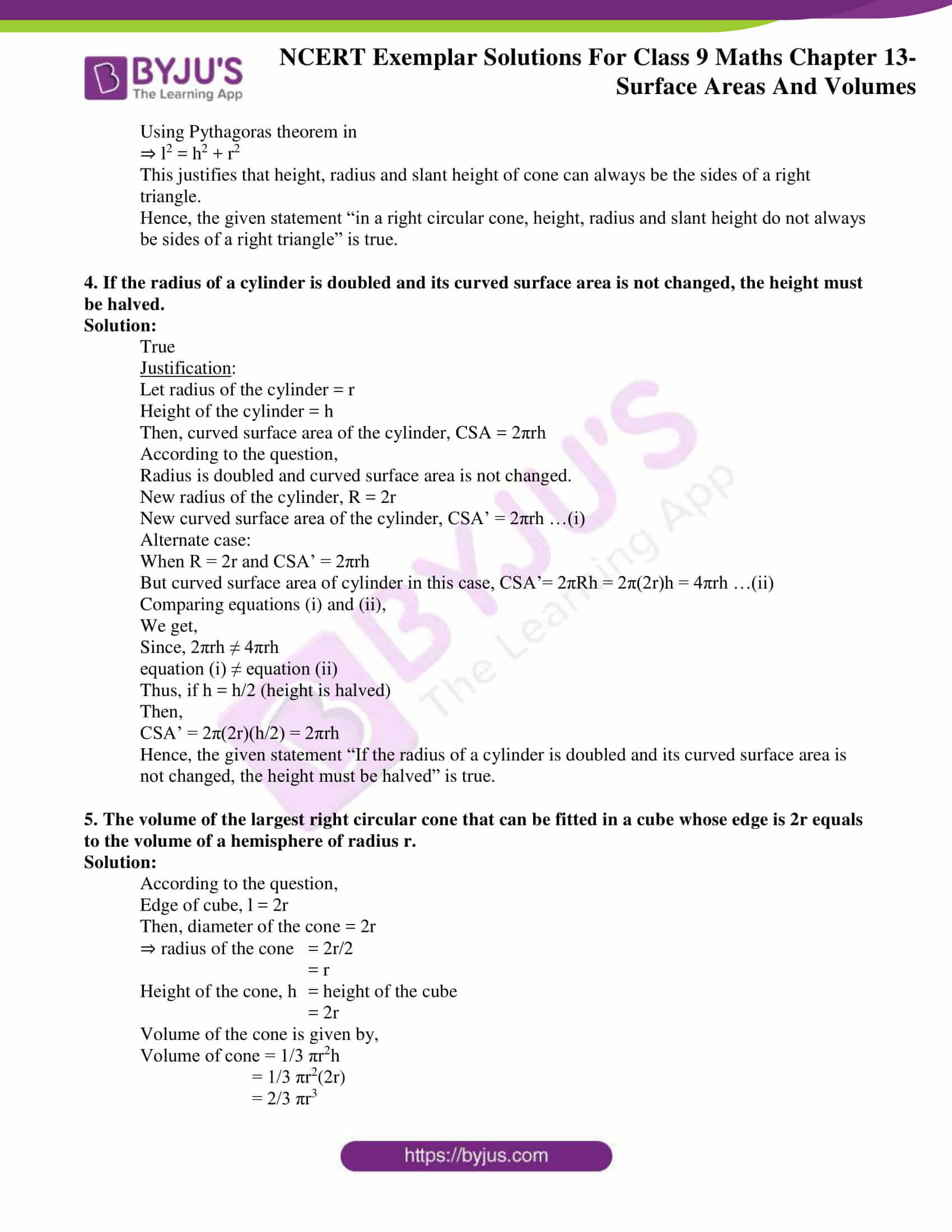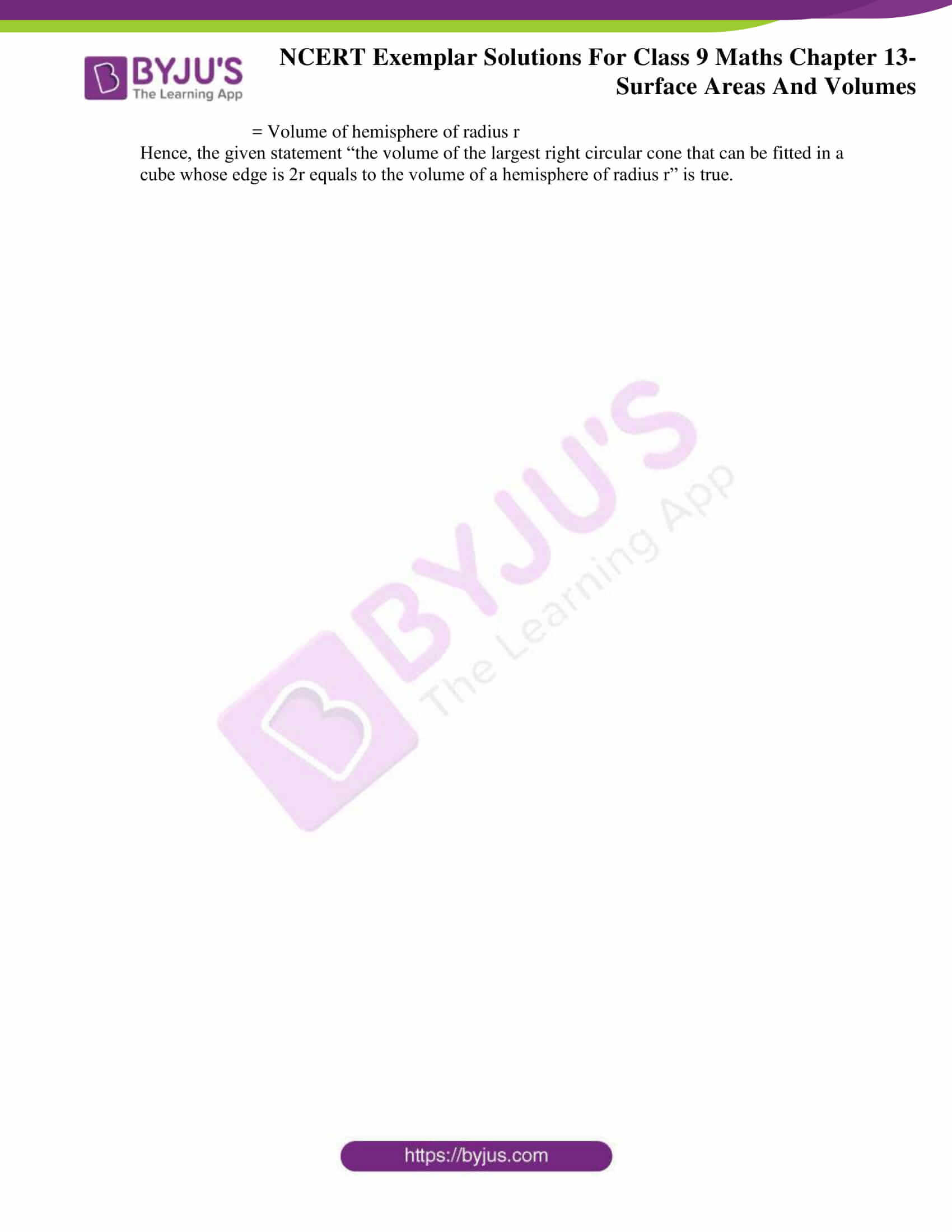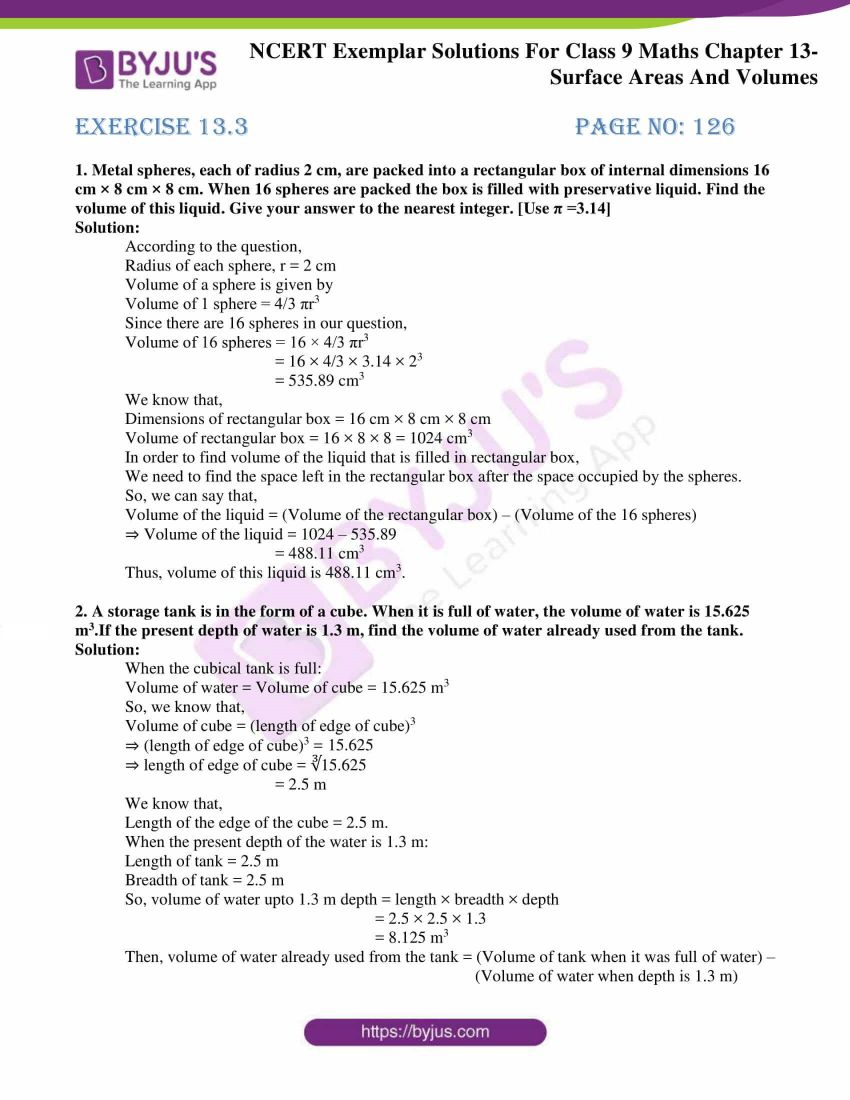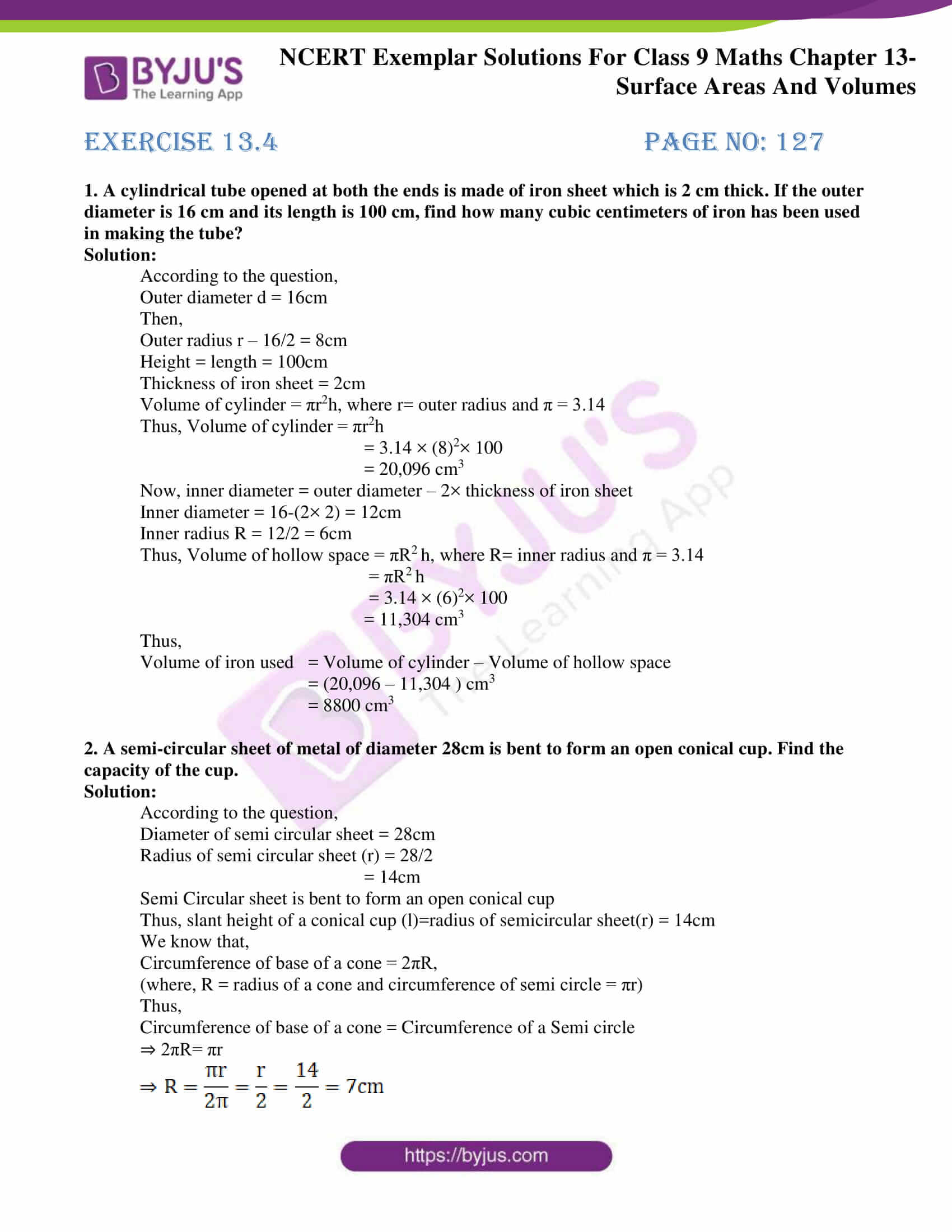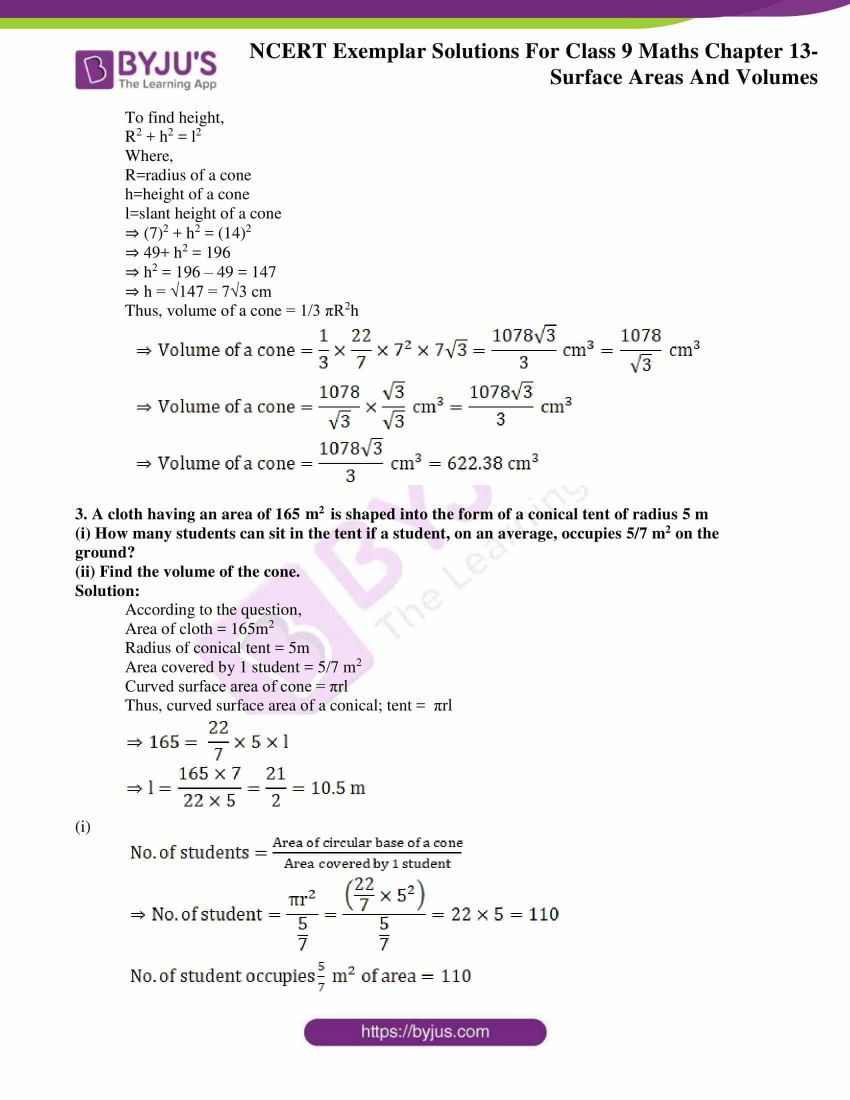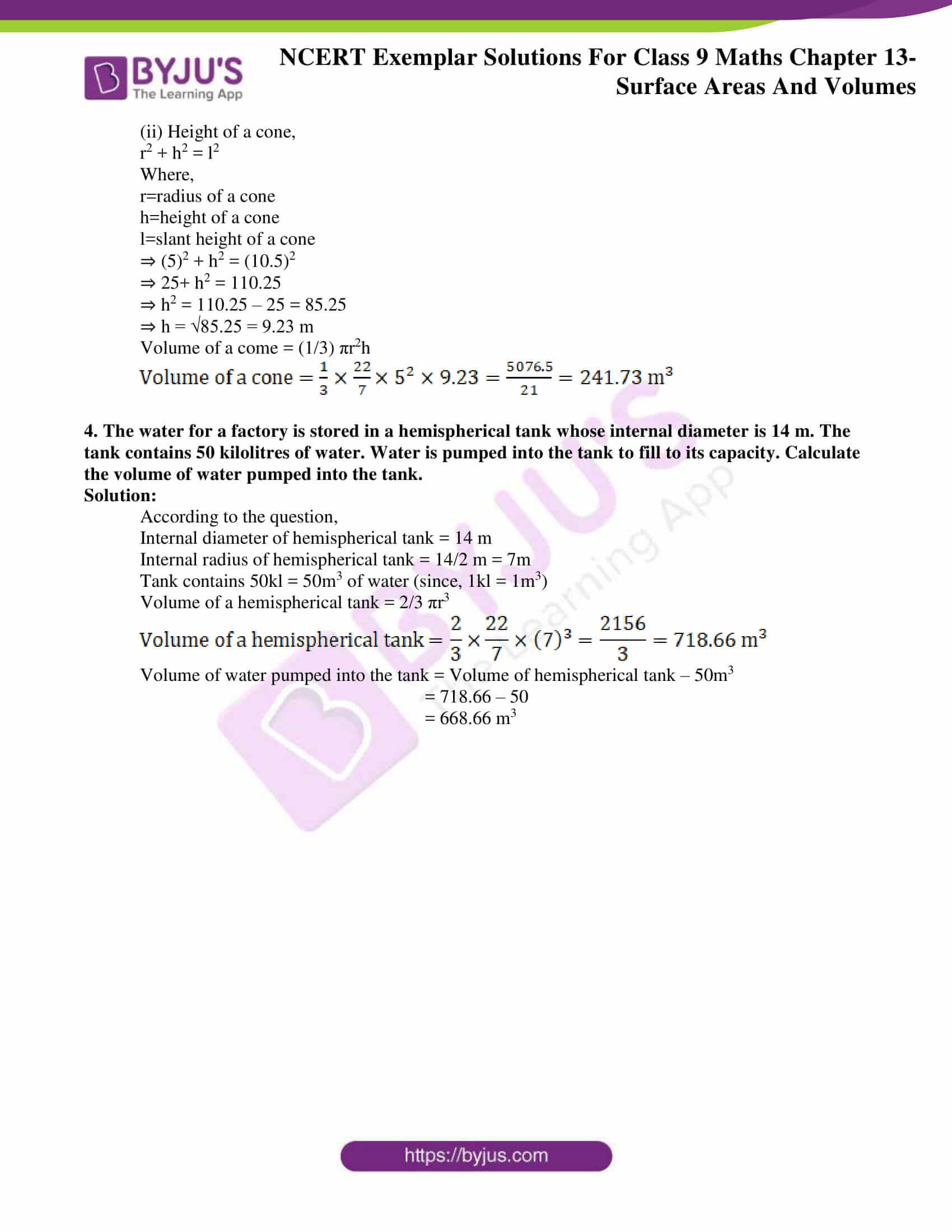### Access answers to NCERT Exemplar Solutions for Class 9 Maths Chapter 13 Surface Areas and Volumes

Exercise 13.1 Page No: 122

Write the correct answer in each of the following:

1. The radius of a sphere is 2r, then its volume will be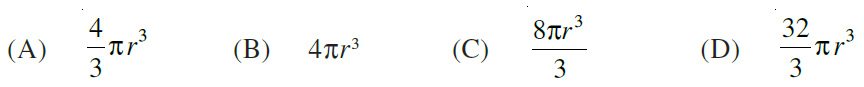Solution:

(D) (32πr3)/3

Explanation:

Given, the radius of a sphere is 2r.

The volume of a sphere = (4/3) × (radius)3

Thus, the volume of the given sphere = (4/3) × π × (2r)3

= (4/3) × π × 8 × r3

= (32 π r3/3)

Hence, option D is the correct answer.

2. The total surface area of a cube is 96 cm2. The volume of the cube is:

(A) 8 cm3 (B) 512 cm3 (C) 64 cm3 (D) 27 cm3

Solution:

(C) 64 cm3

Explanation:

The surface area of a cube is 96 cm2

Let the length of the cube is l cm.

Thus,

6 × l2 = 96

According to formula

l2 = 96/6

l2 = 16

l = 4

Thus the length of the cube is 4 cm.

Volume of a cube  = l3

= 43

= 64

Thus volume is 64 cm3 which is option c.

Hence, option C is the correct answer.

3. A cone is 8.4 cm high and the radius of its base is 2.1 cm. It is melted and recast into a sphere. The radius of the sphere is:

(A) 4.2 cm (B) 2.1 cm (C) 2.4 cm (D) 1.6 cm

Solution:

(B) 2.1 cm

Explanation:

Height of cone, h = 8.4cm

Radius of base, r = 2.1cm

Thus volume of a cone = (1/3) × π × h × r2

=(1/3) × π × 8.4 × (2.1)2

Now when it is melted to form a sphere, say of radius r1 cm, the volumes of both are going to be equal.

Volume of sphere = (4/3) × π × r13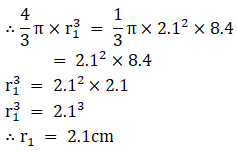Thus the radius of the sphere is 2.1cm which is option b.

Hence, option B is the correct answer.

4. In a cylinder, radius is doubled and height is halved, curved surface area will be

(A) halved (B) doubled (C) same (D) four times

Solution:

(C) same

Explanation:

Let the radius of a cylinder = r unit

Let the height of a cylinder = h unit.

According to question,

Height is halved = h/2

Then,

Curved surface area of a cylinder = 2 × π × r × h

And according to the above condition,

Curved surface area = 2 × π × 2 × r × h/2

= 2 × π × r × h

This is same as the curved surface area of the cylinder with radius r and height h.

Hence, option C is the correct answer.

5. The total surface area of a cone whose radius is r/2 and slant height 2l is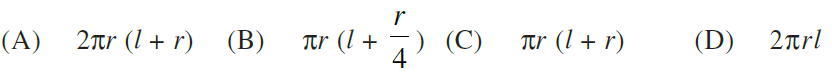Solution:

(B) πr(l+(r/4))

Explanation:

Given, radius of a cone is r/2 and slant height is 2L.

Total surface area = π × radius × slant height + π × radius2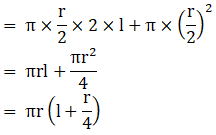Hence, option B is the correct answer

Exercise 13.2 Page No: 124

Write True or False and justify your answer in each of the following:

1. The volume of a sphere is equal to two-third of the volume of a cylinder whose height and diameter are equal to the diameter of the sphere.

Solution:

True

Justification:

Let the radius of the sphere = r.

According to the question,

height and diameter of cylinder = diameter of sphere.

So, the radius of the cylinder = r

And, the height of the cylinder = 2r

We know that,

Volume of sphere = 2/3 volume of cylinder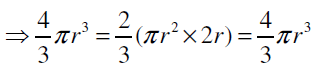Hence, the given statement “the volume of a sphere is equal to two-third of the volume of a cylinder whose height and diameter are equal to the diameter of the sphere” is true.

2. If the radius of a right circular cone is halved and height is doubled, the volume will remain unchanged.

Solution:

False

Justification:

Let the original radius of the cone = r

Let height of the cone = h.

The volume of cone = 1/3 πr2h

Now, when radius of a height circular cone is halved and height is doubled, then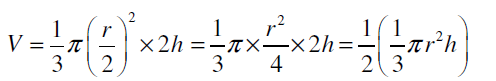We can observe that the new volume = half of the original volume.

Hence, the given statement “if the radius of a right circular cone is halved and height is doubled, the volume will remain unchanged” is false.

3. In a right circular cone, height, radius and slant height do not always be sides of a right triangle.

Solution:

Consider a right circular cone, with

Height = h

And slant height = l

We know, right triangle = one angle 90°

Using Pythagoras theorem in

⇒ l2 = h2 + r2

This justifies that height, radius and slant height of cone can always be the sides of a right triangle.

Hence, the given statement “in a right circular cone, height, radius and slant height do not always be sides of a right triangle” is true.

4. If the radius of a cylinder is doubled and its curved surface area is not changed, the height must be halved.

Solution:

True

Justification:

Let radius of the cylinder = r

Height of the cylinder = h

Then, curved surface area of the cylinder, CSA = 2πrh

According to the question,

Radius is doubled and curved surface area is not changed.

New radius of the cylinder, R = 2r

New curved surface area of the cylinder, CSA’ = 2πrh …(i)

Alternate case:

When R = 2r and CSA’ = 2πrh

But curved surface area of cylinder in this case, CSA’= 2πRh = 2π(2r)h = 4πrh …(ii)

Comparing equations (i) and (ii),

We get,

Since, 2πrh ≠ 4πrh

equation (i) ≠ equation (ii)

Thus, if h = h/2 (height is halved)

Then,

CSA’ = 2π(2r)(h/2) = 2πrh

Hence, the given statement “If the radius of a cylinder is doubled and its curved surface area is not changed, the height must be halved” is true.

5. The volume of the largest right circular cone that can be fitted in a cube whose edge is 2r equals to the volume of a hemisphere of radius r.

Solution:

According to the question,

Edge of cube, l = 2r

Then, diameter of the cone = 2r

⇒ radius of the cone = 2r/2

= r

Height of the cone, h = height of the cube

= 2r

Volume of the cone is given by,

Volume of cone = 1/3 πr2h

= 1/3 πr2(2r)

= 2/3 πr3

= Volume of hemisphere of radius r

Hence, the given statement “the volume of the largest right circular cone that can be fitted in a cube whose edge is 2r equals to the volume of a hemisphere of radius r” is true.

Exercise 13.3 Page No: 126

1. Metal spheres, each of radius 2 cm, are packed into a rectangular box of internal dimensions 16 cm × 8 cm × 8 cm. When 16 spheres are packed the box is filled with preservative liquid. Find the volume of this liquid. Give your answer to the nearest integer. [Use π =3.14]

Solution:

According to the question,

Radius of each sphere, r = 2 cm

Volume of a sphere is given by

Volume of 1 sphere = 4/3 πr3

Since there are 16 spheres in our question,

Volume of 16 spheres = 16 × 4/3 πr3

= 16 × 4/3 × 3.14 × 23

= 535.89 cm3

We know that,

Dimensions of rectangular box = 16 cm × 8 cm × 8 cm

Volume of rectangular box = 16 × 8 × 8 = 1024 cm3

In order to find volume of the liquid that is filled in rectangular box,

We need to find the space left in the rectangular box after the space occupied by the spheres.

So, we can say that,

Volume of the liquid = (Volume of the rectangular box) – (Volume of the 16 spheres)

⇒ Volume of the liquid = 1024 – 535.89

= 488.11 cm3

Thus, volume of this liquid is 488.11 cm3.

2. A storage tank is in the form of a cube. When it is full of water, the volume of water is 15.625 m3.If the present depth of water is 1.3 m, find the volume of water already used from the tank.

Solution:

When the cubical tank is full:

Volume of water = Volume of cube = 15.625 m3

So, we know that,

Volume of cube = (length of edge of cube)3

⇒ (length of edge of cube)3 = 15.625

⇒ length of edge of cube = ∛15.625

= 2.5 m

We know that,

Length of the edge of the cube = 2.5 m.

When the present depth of the water is 1.3 m:

Length of tank = 2.5 m

Breadth of tank = 2.5 m

So, volume of water upto 1.3 m depth = length × breadth × depth

= 2.5 × 2.5 × 1.3

= 8.125 m3

Then, volume of water already used from the tank = (Volume of tank when it was full of water) –

(Volume of water when depth is 1.3 m)

= 15.625 – 8.125

= 7.5 m3

Thus, volume of water already used from the tank is 7.5 m3.

3. Find the amount of water displaced by a solid spherical ball of diameter 4.2 cm, when it is completely immersed in water.

Solution:

Water displaced when a solid spherical ball is immersed completely in water equals to its own volume.

According to the question,

Diameter of spherical ball = 4.2 cm

⇒ radius of spherical ball = 4.2/2 = 2.1 cm

So, volume of a sphere = 4/3 πr3

(4/3) (22/7)(2.1)3 = 38.81

Thus, volume of water displaced is 38.81 cm3.

4. How many square metres of canvas is required for a conical tent whose height is 3.5 m and the radius of the base is 12 m?

Solution:

According to the question,

Dimensions of conical tent are:

Height = 3.5 m

Curved surface area of cone = πr√(r2 + h2)

= 22/7 × 12 √(122 + 3.52)

= 22/7 × 12 × √156.25

= 22/7 × 12 × 12.5

= 471.43 m2

Since, area of canvas = curved surface area of conical tent

Therefore, area of canvas required is 471.43 m2.

Exercise 13.4 Page No: 127

1. A cylindrical tube opened at both the ends is made of iron sheet which is 2 cm thick. If the outer diameter is 16 cm and its length is 100 cm, find how many cubic centimeters of iron has been used in making the tube?

Solution:

According to the question,

Outer diameter d = 16cm

Then,

Outer radius r – 16/2 = 8cm

Height = length = 100cm

Thickness of iron sheet = 2cm

Volume of cylinder = πr2h, where r= outer radius and π = 3.14

Thus, Volume of cylinder = πr2h

= 3.14 × (8)2× 100

= 20,096 cm3

Now, inner diameter = outer diameter – 2× thickness of iron sheet

Inner diameter = 16-(2× 2) = 12cm

Inner radius R = 12/2 = 6cm

Thus, Volume of hollow space = πRh, where R= inner radius and π = 3.14

= πRh

= 3.14 × (6)2× 100

= 11,304 cm3

Thus,

Volume of iron used = Volume of cylinder – Volume of hollow space

= (20,096 – 11,304 ) cm3

= 8800 cm3

2. A semi-circular sheet of metal of diameter 28cm is bent to form an open conical cup. Find the capacity of the cup.

Solution:

According to the question,

Diameter of semi circular sheet = 28cm

Radius of semi circular sheet (r) = 28/2

= 14cm

Semi Circular sheet is bent to form an open conical cup

Thus, slant height of a conical cup (l)=radius of semicircular sheet(r) = 14cm

We know that,

Circumference of base of a cone = 2πR,

(where, R = radius of a cone and circumference of semi circle = πr)

Thus,

Circumference of base of a cone = Circumference of a Semi circle

⇒ 2πR= πr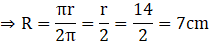To find height,

R2 + h2 = l2

Where,

h=height of a cone

l=slant height of a cone

⇒ (7)2 + h2 = (14)2

⇒ 49+ h2 = 196

⇒ h2 = 196 – 49 = 147

⇒ h = √147 = 7√3 cm

Thus, volume of a cone = 1/3 πR2h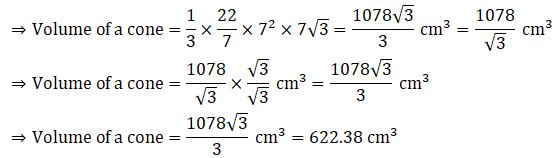3. A cloth having an area of 165 m2 is shaped into the form of a conical tent of radius 5 m

(i) How many students can sit in the tent if a student, on an average, occupies 5/7 m2 on the ground?

(ii) Find the volume of the cone.

Solution:

According to the question,

Area of cloth = 165m2

Radius of conical tent = 5m

Area covered by 1 student = 5/7 m2

Curved surface area of cone = πrl

Thus, curved surface area of a conical; tent =  πrl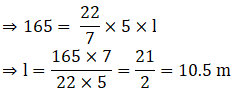(i)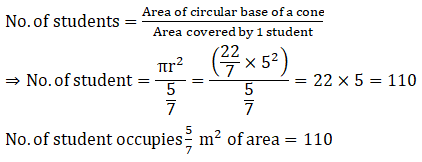(ii) Height of a cone,

r2 + h2 = l2

Where,

h=height of a cone

l=slant height of a cone

⇒ (5)2 + h2 = (10.5)2

⇒ 25+ h2 = 110.25

⇒ h2 = 110.25 – 25 = 85.25

⇒ h = √85.25 = 9.23 m

Volume of a come = (1/3) πr2h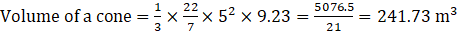4. The water for a factory is stored in a hemispherical tank whose internal diameter is 14 m. The tank contains 50 kilolitres of water. Water is pumped into the tank to fill to its capacity. Calculate the volume of water pumped into the tank.

Solution:

According to the question,

Internal diameter of hemispherical tank = 14 m

Internal radius of hemispherical tank = 14/2 m = 7m

Tank contains 50kl = 50m3 of water (since, 1kl = 1m3)

Volume of a hemispherical tank = 2/3 πr3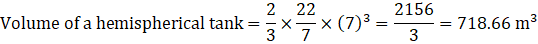Volume of water pumped into the tank = Volume of hemispherical tank – 50m3

= 718.66 – 50

= 668.66 m3

Download BYJU’S-The Learning App and get personalized videos, explaining different types of Maths topics such as surface areas and volumes of solids, etc. and experience a new way of learning to understand the concepts easily.

## Frequently Asked Questions on NCERT Exemplar Solutions for Class 9 Maths Chapter 13

### What is an effective way to study Chapter 13 of NCERT Exemplar Solutions for Class 9 Maths?

The problems and solutions of Chapter 13 Surface areas and volumes play an important role in building a strong foundation of fundamental concepts. The below mentioned steps should be followed in order to cover the concepts effectively-
1. Glance the basic concepts
2. Understand the topic of surface areas
3. Memorise the important formulas
4. Learn all the formulas
5. Practice the problems on a daily basis.

### What are the topics included in the Chapter 13 of NCERT Exemplar Solutions for Class 9 Maths?

Students must first understand that the topics included in Chapter 13 of NCERT Solutions for Class 11 Maths creates a strong foundation of surface areas and volumes. The topics covered in this chapter are –
1. Formulas for Surface Area of a Cuboid and a Cube
2. Formulas for Surface Area(curved surface and total surface) of a Right Circular Cylinder
3. Curved surface area and total surface area of a cone
4. The surface area of a sphere
5. Curved Surface Area and Total Surface Area of a Hemisphere
6. Volumes of Cuboid, Cylinder, Right Circular Cone, Sphere and Hemisphere.

### Explain the area of the hollow hemisphere as per the Chapter 13 of NCERT Exemplar Solutions for Class 9 Maths.

A hollow hemisphere has two diameters for its circular bases, one is for the inside circular base (hollow part) and one is for the outside circular base (rigid part). Therefore, the area of the hollow hemisphere is equal to the difference between the area of the external hemisphere and the area of the internal hemisphere.
Area of Hollow Hemisphere = Area of External Hemisphere – Area of Internal Hemisphere
Students must keep this topic in mind while studying this chapter which will help them to learn the concepts faster.